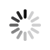#### Welcome!

×Forgot password?
Go back to Challenges list

Challenge

## Type of

Your code should analyse a given string so that you can identify the type of each "part" of the string.
To analyse this string you should first split this string by " " (spaces). If some of this parts is equal to " " (blank space or 1 space), it should be deleted. After it, you should verify if each part correspond to one of this types:
• Array
• Integer Number
• Floating-point Number
• String
The output should be something like this: "Found X arrays, X strings, X integer numbers and X floating-point numbers." (without quote marks).
To identify this correctly, there are some rules for each type.

###### Array Rules:
• The only notation accepted is [...]. Any other is classified a string.
• Arrays of arrays are not counted.
• Blank arrays are not accepted and are classified as a string.
• You can use any division inside arrays but spaces are not permitted.
• The items that are into the array should not be analyzed for the final result.
• Arrays can have one point after "]" if the array is the last thing of the sentence.

"[array,[of,array]]" Found 1 arrays, 0 strings, 0 integer numbers and 0 floating-point numbers. The array inside the array is not analysed.
"[asa,corta] 5" Found 1 arrays, 0 strings, 1 integer numbers and 0 floating-point numbers. Array: "[asa,corta]" Integer: "5"
"[asa, corta]" Found 0 arrays, 2 strings, 0 integer numbers and 0 floating-point numbers. Strings: "[asa," and "corta]"
"{bread,5}" Found 0 arrays, 1 strings, 0 integer numbers and 0 floating-point numbers. String: "{bread,5}"
"[]" Found 0 arrays, 1 strings, 0 integer numbers and 0 floating-point numbers. String: "[]"
"[first/2nd/third]" Found 1 arrays, 0 strings, 0 integer numbers and 0 floating-point numbers. Array: "[first/2nd/third]"

###### Integer Number / Floating-point number
• Positive and negative numbers are permitted.
• Can have one point after it if it is the last thing of the sentence.

"This is my sentence n. 5" Found 0 arrays, 5 strings, 1 integer numbers and 0 floating-point numbers. Strings: "This", "is", "my", "sentence", "n." Integer: "5"
"+5 -4.2" Found 0 arrays, 0 strings, 1 integer numbers and 1 floating-point numbers. Integer: "+5" Float: "-4.2"
"Hello. I am 14." Found 0 arrays, 3 strings, 1 integer numbers and 0 floating-point numbers. Strings: "Hello.", "I", "am" Integer number: "14" (point is ignored because it is the last item of the phrase)

###### Floating-point number
• The number should use this format: 0-9.0-9 or 0.9,0.9 . Something else is considered a string.
"1.2.3" Found 0 arrays, 1 strings, 0 integer numbers and 0 floating-point numbers. String: "1.2.3"
"1.2 word/)/=)?(/(UIJBHVGYUHNJ" Found 0 arrays, 1 strings, 0 integer numbers and 1 floating-point numbers. String: "word/)/=)?(/(UIJBHVGYUHNJ" Float: "1.2"
".2 Hi" Found 0 arrays, 2 strings, 0 integer numbers and 0 floating-point numbers. String: ".2", "Hi"
".2 Hi" Found 0 arrays, 2 strings, 0 integer numbers and 0 floating-point numbers. String: ".2", "Hi"

###### String
• Is everything that is not an array, integer number or floating-point number.

###### Sample Input
```I've bought 5 [bread,apples,pears]. If pi is 3.14059 I'm unpi.```

###### Sample Output
```Found 1 arrays, 2 strings, 1 integer numbers and 0 floating-point numbers. Found 0 arrays, 5 strings, 0 integer numbers and 1 floating-point numbers.```

`512M`